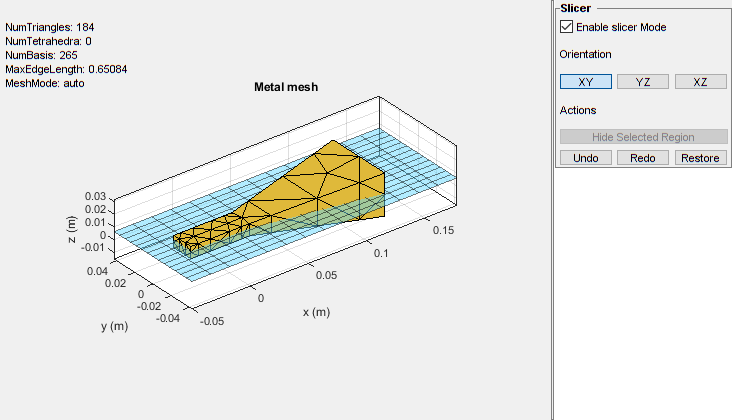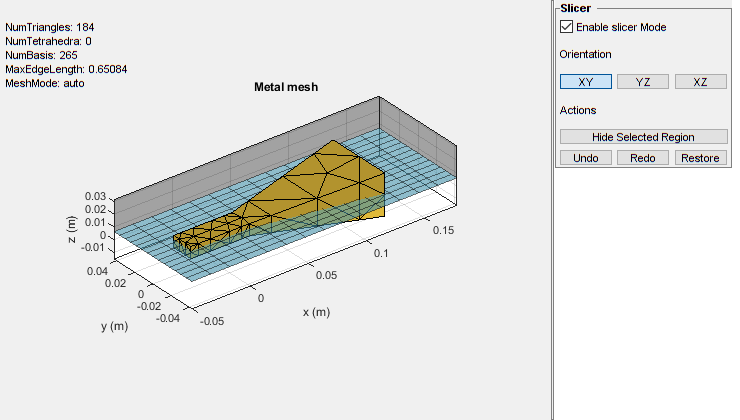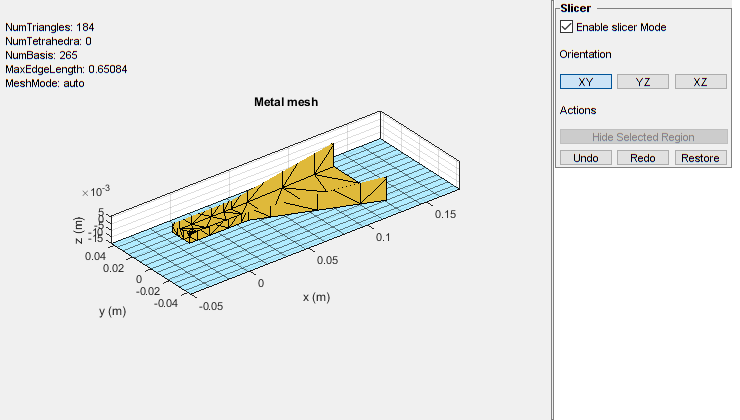# mesh

Mesh properties of metal, dielectric antenna, or array structure

## Syntax

``mesh(object)``
``mesh(shape)``
``mesh(object,Name=Value)``
``meshdata = mesh(___,Name=Value)``

## Description

````mesh(object)` plots the mesh that is used to analyze the specified antenna or array element.```

example

````mesh(shape)` plots the mesh for the specified shapes.```

example

````mesh(object,Name=Value)` specifies additional options using name-value arguments.```

example

````meshdata = mesh(___,Name=Value)` returns the mesh as a structure that contains the properties used to analyze the antenna or array. Use this syntax to determine the number of basis functions in the output.```

## Examples

collapse all

Create and view the mesh structure of a top-hat monopole antenna with Maximum edge length of 0.1 m.

```h = monopoleTopHat; i = impedance(h,75e6)```
```i = 2.4127e+02 + 5.8817e+02i ```
`mesh(h)``m = mesh(h)`
```m = struct with fields: NumTriangles: 148 NumTetrahedra: 0 NumBasis: 203 MaxEdgeLength: 0.4295 MinEdgeLength: 0.3221 GrowthRate: 0.9500 MeshMode: 'auto' ```

Radiation Pattern of Microstrip Patch Antenna

Create a microstrip patch antenna using 'FR4' as the dielectric substrate.

```d = dielectric('FR4'); pm = patchMicrostrip(Length=75e-3,Width=37e-3,... GroundPlaneLength=120e-3,GroundPlaneWidth=120e-3,... Substrate=d); show(pm)```Plot the radiation pattern of the antenna at a frequency of 1.67 GHz.

```figure pattern(pm,1.67e9)```Mesh the whole antenna.

```figure mesh(pm)```Mesh only the dielectric surface of the antenna.

```figure mesh(pm,View='dielectric surface')```Create a rectangular and circular shape, intersect them and mesh at a wavelength of 2 m.

```r = antenna.Rectangle; c = antenna.Circle; p = r&c; mesh(p,2);```Create a default horn antenna. Mesh the structure with the `'Slicer'` argument set to `'on'`.

```ant = horn; z = impedance(ant,70e6); mesh(ant,Slicer='on')```Select Enable slicer Mode. Then select a plane for the slice under Orientation.Click on the plot and select a region to hide.Click Hide Selected Region to view the desired slice.## Input Arguments

collapse all

Antenna or array element, specified as an object.

Example: `ant = dipole; mesh(ant)`

Shape created using custom elements and shape objects of Antenna Toolbox, specified as an object. You can create the shapes using `antenna.Circle`, `antenna.Polygon`, or `antenna.Rectangle`.

Example: `c = antenna.Rectangle; mesh(c)`

### Name-Value Arguments

Specify optional pairs of arguments as `Name1=Value1,...,NameN=ValueN`, where `Name` is the argument name and `Value` is the corresponding value. Name-value arguments must appear after other arguments, but the order of the pairs does not matter.

Before R2021a, use commas to separate each name and value, and enclose `Name` in quotes.

Example: `MaxEdgeLength=0.1`

Maximum edge length of triangles in mesh, specified as a comma-separated pair consisting of `'MaxEdgeLength'` and a scalar in meters.

Example: `MaxEdgeLength=0.1`

Data Types: `double`

Smallest edge length of the triangles in the mesh, specified as a positive scalar in meters.

Example: `MinEdgeLength=0.1`

Data Types: `double`

Gradation in the triangle sizes of the mesh, specified as a scalar in the range (0, 1).

Example: `GrowthRate=0.7` The value `0.7` states that the growth rate of the mesh is 70 percent.

Data Types: `double`

Customize mesh view of antenna or array element, specified as a comma-separated pair consisting of `'View'` and `'all'`, `'metal'`,`'dielectric surface'`, or `'dielectric volume'`.

You choose `'dielectric surface'` to view the boundary triangle mesh of the dielectric. You choose `'dielectric volume'` to view the tetrahedral volume mesh of the dielectric.

Example: `View='metal'`

Data Types: `char`

Option to enable or disable plot interactivity, specified as `'on'` or `'off'`, or as numeric or logical `1(true)` or `0(false)`. Set this argument to `1` or `'on'` to open the plot with the slicer panel, and to slice and view the desired cross section of the plot along the xy-, yz-, and xz- planes. Set this argument to `0` or `'off'` to open the plot without the slicer panel.

Example: `Slicer='on'`

Data Types: `string` | `logical`

## Version History

Introduced in R2015a